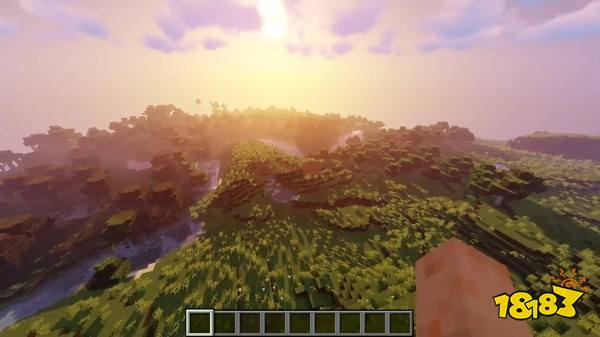我的世界地图种子 结构与群系分布{

int i = chunkX;

int j = chunkZ;

if (chunkX < 0)

{

chunkX -= this.distance - 1;

}

if (chunkZ < 0)

{

chunkZ -= this.distance - 1;

}

int k = chunkX / this.distance;

int l = chunkZ / this.distance;

Random random = this.world.setRandomSeed(k, l, 10387312);//LINE_1

k = k * this.distance;

l = l * this.distance;

k = k + random.nextInt(this.distance - 8);

l = l + random.nextInt(this.distance - 8);

if (i == k && j == l)

{

boolean flag = this.world.getBiomeProvider().areBiomesViable(i * 16 + 8, j * 16 + 8, 0, VILLAGE_SPAWN_BIOMES);//LINE_2

if (flag)

{

return true;

}

}

return false;

}

相关推荐+  更多礼包+  更多

• 我的世界中国版18183豪华礼包 领取
• 我的世界18183蓝宝石礼包 领取
• 我的世界中国版点卡抽奖礼包 领取
• 我的世界中国版Q币活动礼包（长期） 领取
• 我的世界感恩节福利活动礼包 领取
• 我的世界双十一有奖活动礼包 领取
• 我的世界万圣节恶搞活动礼包 领取
• 我的世界十月新版本活动礼包 领取

原创攻略+  更多18183手游网